# 搜索引擎如何推荐网页dongge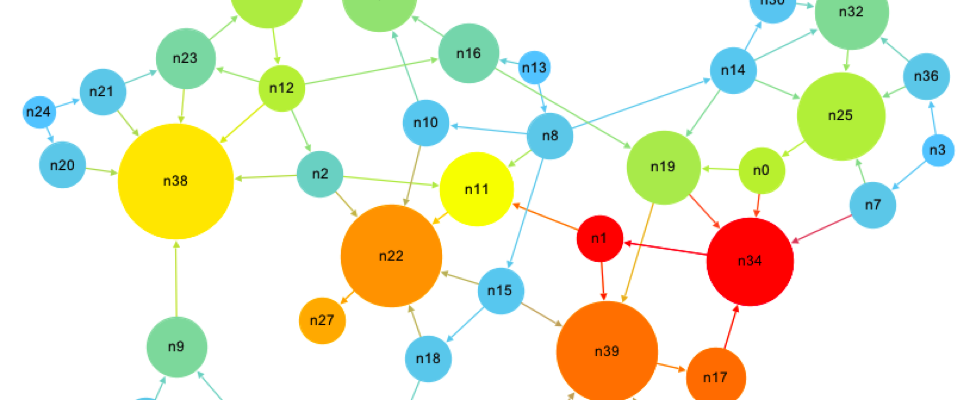## PageRank 与矩阵的关系

﻿﻿﻿﻿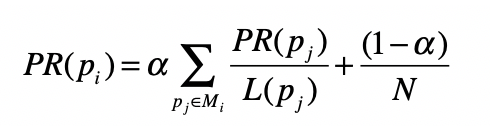﻿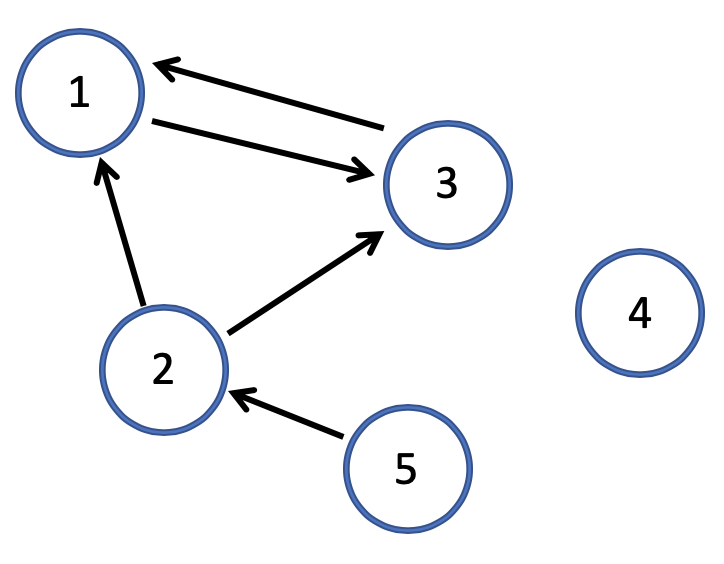﻿

﻿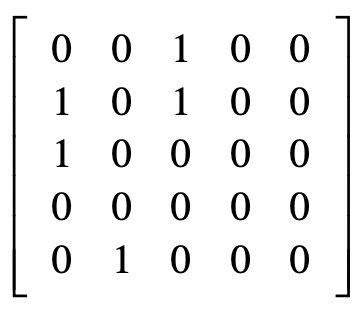﻿

﻿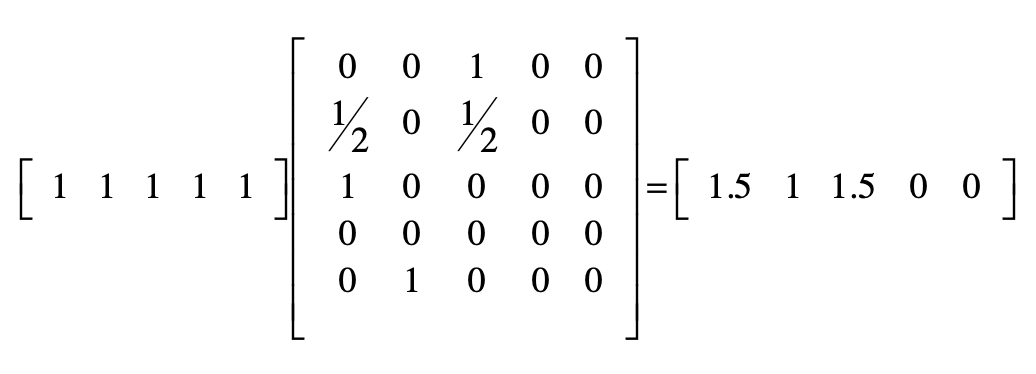﻿﻿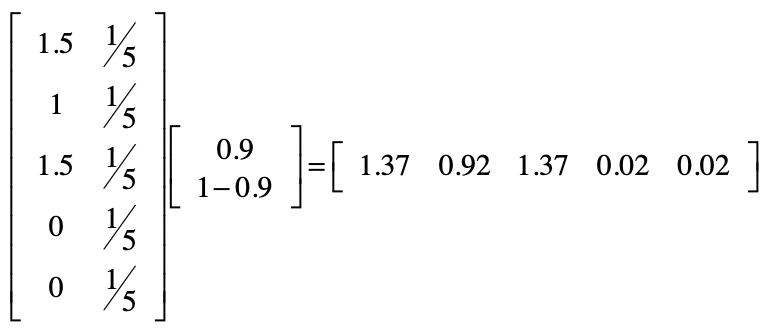﻿

[0.37027027 0.24864865 0.37027027 0.00540541 0.00540541]

## PageRank 的程序实现

import numpy as np# 设置确定随机跳转概率的alpha、网页结点数alpha = 0.9N = 5# 初始化随机跳转概率的矩阵jump = np.full([2,1], [[alpha], [1-alpha]], dtype=float)# 邻接矩阵的构建adj = np.full([N,N], [[0,0,1,0,0],[1,0,1,0,0],[1,0,0,0,0],[0,0,0,0,0],[0,1,0,0,0]], dtype=float)# 对邻接矩阵进行归一化row_sums = adj.sum(axis=1)      # 对每一行求和row_sums[row_sums == 0] = 0.1   # 防止由于分母出现0而导致的Nanadj = adj / row_sums[:, np.newaxis] # 除以每行之和的归一化# 初始的PageRank值，通常是设置所有值为1.0pr = np.full([1,N], 1, dtype=float)

﻿

# PageRank算法本身是采样迭代方式进行的，当最终的取值趋于稳定后结束。for i in range(0, 20):    # 进行点乘，计算Σ(PR(pj)/L(pj))    pr = np.dot(pr, adj)    # 转置保存Σ(PR(pj)/L(pj))结果的矩阵，并增加长度为N的列向量，其中每个元素的值为1/N，便于下一步的点乘。    pr_jump = np.full([N, 2], [[0, 1/N]])    pr_jump[:,:-1] = pr.transpose()    # 进行点乘，计算α(Σ(PR(pj)/L(pj))) + (1-α)/N)    pr = np.dot(pr_jump, jump)    # 归一化PageRank得分    pr = pr.transpose()    pr = pr / pr.sum()    print("round", i + 1, pr)

﻿

﻿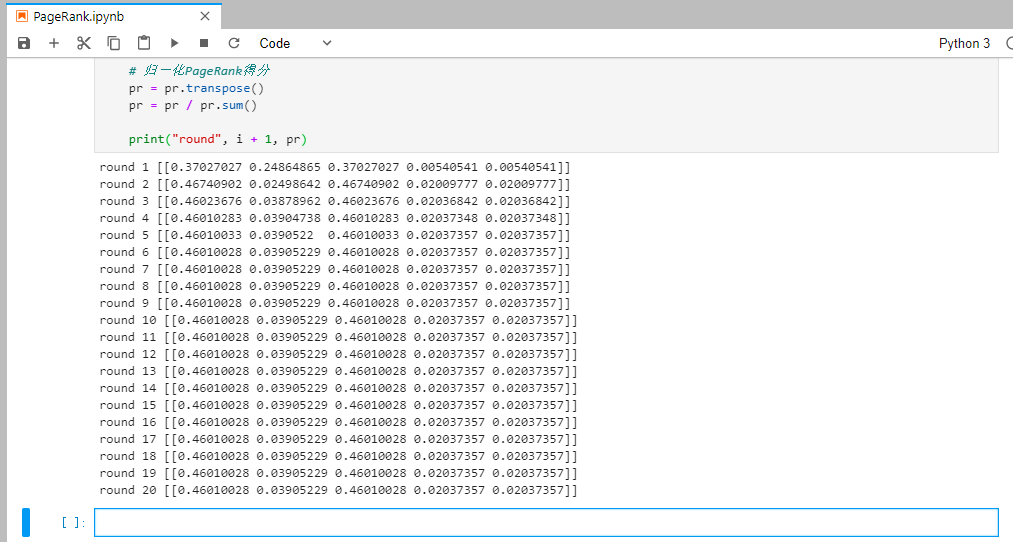﻿

﻿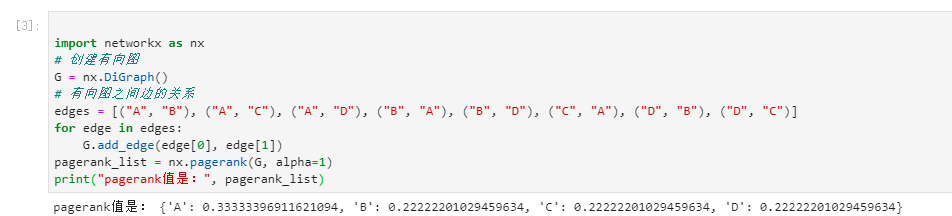﻿

﻿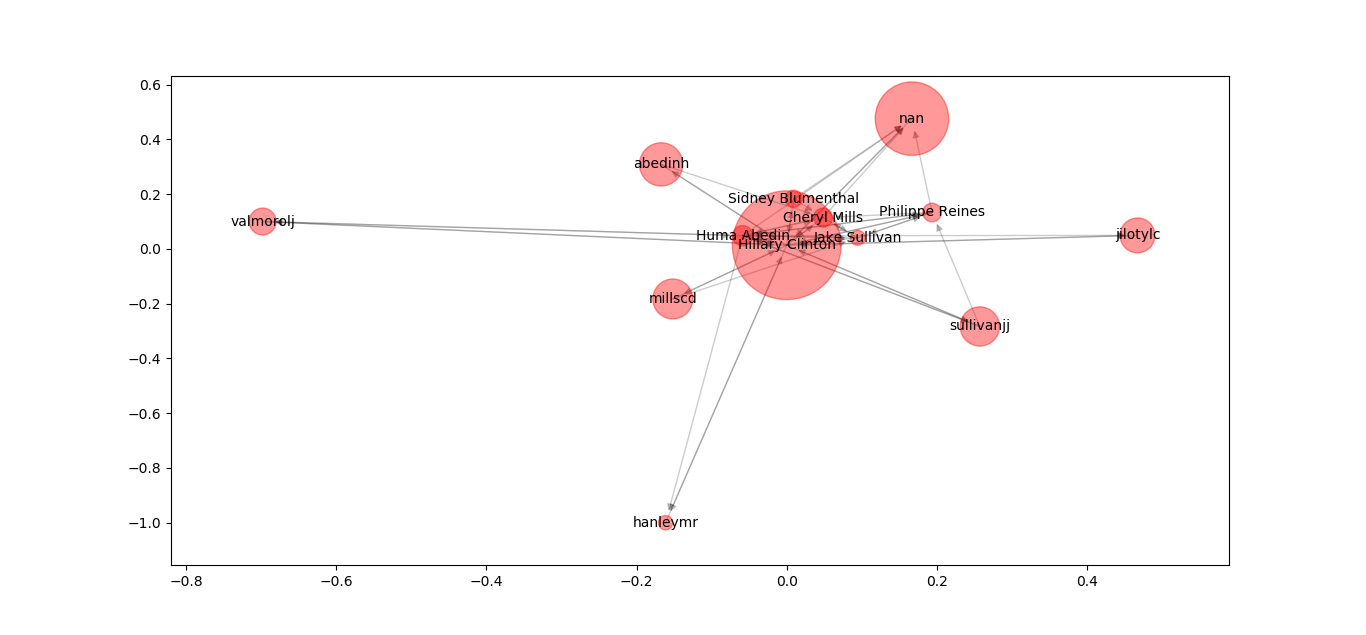﻿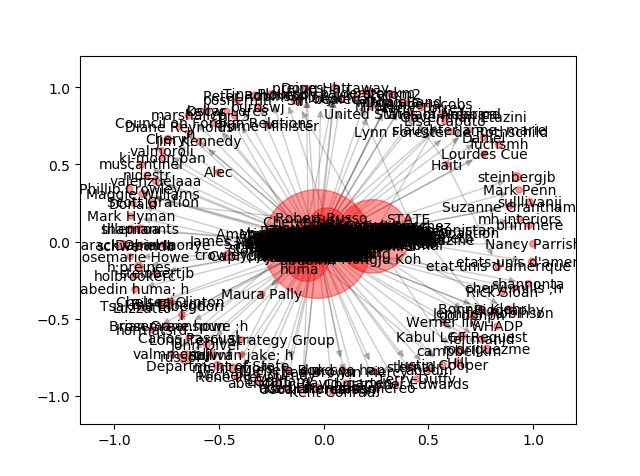﻿

## 参考

https://zh.wikipedia.org/wiki/PageRank

https://time.geekbang.org/column/article/85194

https://time.geekbang.org/column/article/83034

https://time.geekbang.org/column/article/83471

﻿## 评论